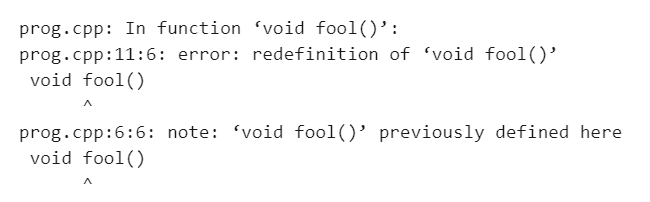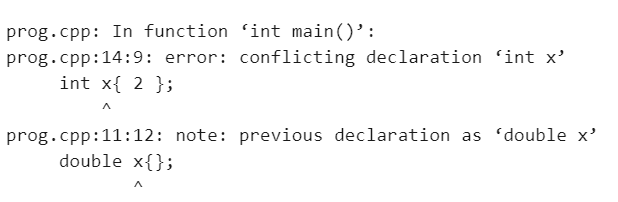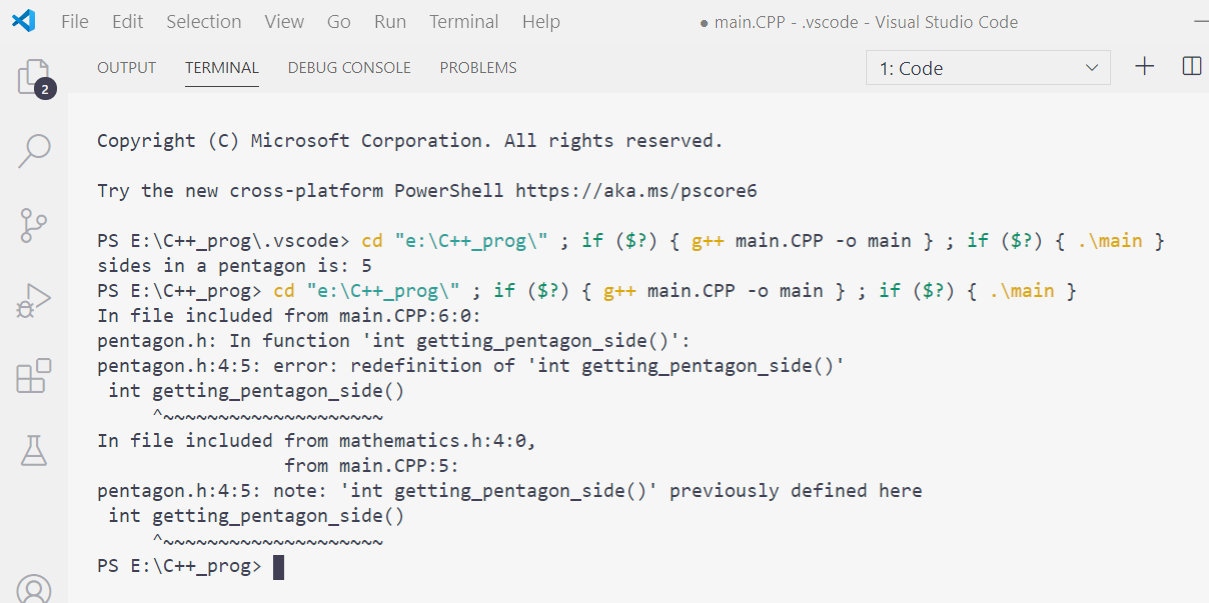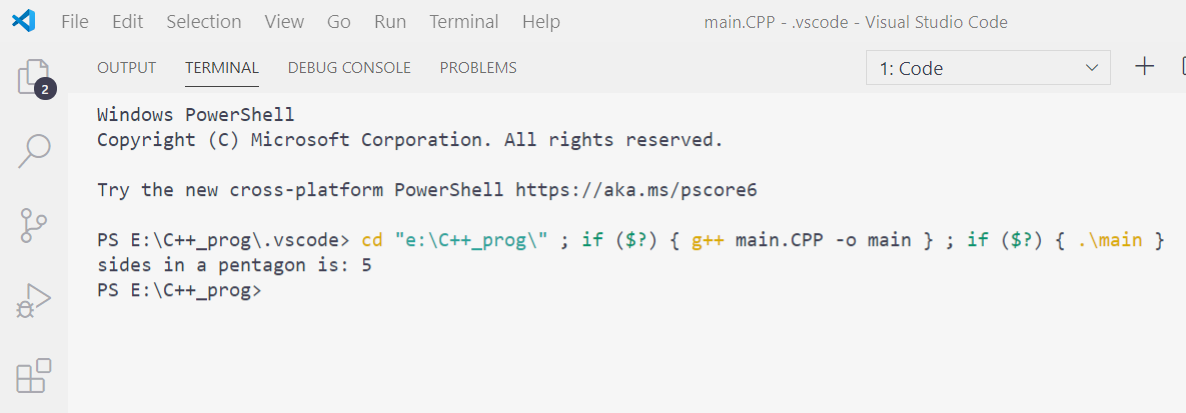Related Articles
• Difficulty Level : Expert
• Last Updated : 02 Nov, 2020

Header Guards in C++ are conditional compilation directives that help to avoid errors that arise when the same function or variable is defined more than once by the mistake of a programmer. According to C++, when a function or a variable is defined more than once, it yields an error. Below is the program to illustrate the same:

Program 1:

## C++

 `// C++ program to illustrate error ` `// caused due to defining same ` `// function more than once ` `#include ` `using` `namespace` `std; ` ` `  `// Function 1 ` `void` `fool() ` `{ ` `    ``cout << ``"hello"``; ` `} ` ` `  `// Function 2 ` `void` `fool() ` `{ ` `    ``cout << ``"hello maddy"``; ` `} ` ` `  `// Driver Code ` `int` `main() ` `{ ` `    ``// Function Call ` `    ``fool(); ` ` `  `    ``return` `0; ` `} `

Output:Explanation:
In the above program, a function fool() has been defined twice which is causing a fatal error.

Program 2:

## C++

 `// C++ program to illustrate error ` `// caused due to defining same ` `// variable more than once ` `#include ` `using` `namespace` `std; ` ` `  `// Driver Code ` `int` `main() ` `{ ` `    ``// error: note: 'double x' previously ` `    ``double` `x{}; ` ` `  `    ``// declared here ` `    ``int` `x{ 2 }; ` ` `  `    ``return` `0; ` `} `

Output:Explanation:
In the above program, the variable x has been defined twice which is causing a fatal error.

These are the most commonly occurring errors and can be fixed with a very basic knowledge of programming. But if a situation arises where a Header file is included in a program and inadvertently a forward declaration of a function is done which has been a part of the already included header file. In that case, the content of the header file is not remembered, no matter whether it’s user-defined or predefined.

So further, a fatal error is encountered which sometimes becomes unresolvable. For this, C++is armed with some preprocessor directives that avoid this error.

• Let there is a header file named pentagon.h as below:

## C++

 `// Function to get the side of ` `// the pentagon ` `int` `getting_pentagon_side() ` `{ ` `    ``// Return the side ` `    ``return` `5; ` `}`

• Let there be another header file named “mathematics.h” that includes the header file “pentagon.h”.

## C++

 `// Including another header file ` `// "pentagon.h" in the current program ` `#include "pentagon.h" `

• Now, a C++ program is created with the name “pentagon.cpp” as shown below:

Program 3:

## C++

 `// C++ program to illustrate the error ` `// discussed above ` `#include ` ` `  `// Include header files created ` `#include "mathematics.h" ` `#include "pentagon.h" ` `using` `namespace` `std; ` ` `  `// Driver Code ` `int` `main() ` `{ ` ` `  `    ``return` `0; ` `} `

Output:To solve the error caused above, the idea is to use the concept of “Header Guard” to avoid errors that occur when the same function variable is defined more than once by the mistake of the programmer.

A header guard can avoid such errors by using Conditional Compilation directives. It is a combination of Conditional Compilation directives that protect your header from being included in a program multiple numbers of times.

Syntax:

```#ifndef HEADER_H_NAME
/*...
...*/
#endif
```

Now, Let’s come to the previous example and see how the error conflict can be resolved:

• First, the “pentagon.h” header file is modified as:
```#ifndef PENTAGON_H
#define PENTAGON_H

int getting_pentagon_side()
{
return 5;
}
#endif

```
• Now the “mathematics.h” header file that includes “pentagon.h” is modified as:
```#ifndef MATHEMATICS_H
#define MATHEMATICS_H
#include "pentagon.h"
#endif

```

Below is the program after the above changes to avoid the errors:

## C++

 `// C++ program to illustrate how to ` `// avoid errors using Header Guard ` `#include ` ` `  `// Include header guards in both ` `// the header files ` `#include "mathematics.h" ` ` `  `// Now, the error will not occur ` `#include "pentagon.h" ` ` `  `using` `namespace` `std; ` ` `  `// Driver Code ` `int` `main() ` `{ ` `    ``// Function Call to find the ` `    ``// sides of the pentagon ` `    ``int` `i{ getting pentagon side() }; ` ` `  `    ``// Print the sides ` `    ``cout << ``"sides in a pentagon is: "` `         ``<< i; ` ` `  `    ``return` `0; ` `} `

Output:Rated as one of the most sought after skills in the industry, own the basics of coding with our C++ STL Course and master the very concepts by intense problem-solving.

My Personal Notes arrow_drop_up
Recommended Articles
Page :